# Light-Emitting Diodes (LEDs)

In some local-area networks, a coherent source is not required, and one can employ a light-emitting diode (LED), a less expensive and longer-lasting optical source with a relatively wide optical spectrum. The basic structure of an LED is similar to that of semiconductor lasers in the sense that both employ an active layer sandwiched between two cladding layers and pumped using a forward-biased p-n junction. The main difference is that stimulations emission does not occur because a population inversion is not realized. Rather, radiative recombination of electron-hole pairs in the active layer generates light through spontaneous emission, some of which escapes from the device and can be coupled into an optical fiber. The emitted light is incoherent with a relatively wide spectral width (30-60 nm) and a relatively large angular spread.

#### 1. Continuous Wave (CW) Characteristics

It is easy to estimate the internal power generated by spontaneous emission. At a given current I the carrier-injection rate is I/q. In the steady state, the rate of electron-hole pairs recombining through radiative and nonradiative processes is equal to the carrier-injection rate I/q. Since the internal quantum efficiency ηint determines the fraction of electron-hole pairs that recombine through spontaneous emission, the rate of photon generation is simply ηintI/q. The internal optical power is thus given by

Pintηint(ћω/q)I

where ћω is the photon energy, assumed to be nearly the same for all photons. If ηext is the fraction of photons escaping from the device, the emitted power is given by

Peηextηint(ћω/q)I

The quantity ηext is called the external quantum efficiency. It can be calculated by taking into account internal absorption and the total internal reflection at the semiconductor-air interface. As seen in the figure below, only light emitted within a cone of angle θc, where θc = sin-1(1/n) is the critical angle and n is the refractive index of the semiconductor material, escapes from the LED surface.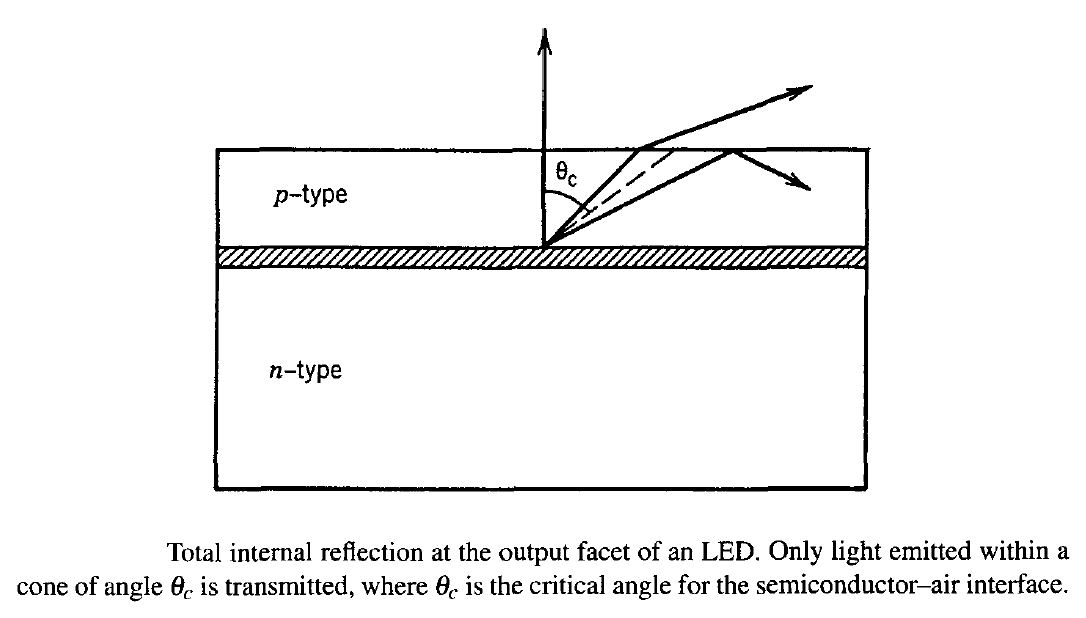Internal absorption can be avoided by using heterostructure LEDs in which the cladding layers surrounding the active layer are transparent to the radiation generated. The external quantum efficiency can then be written as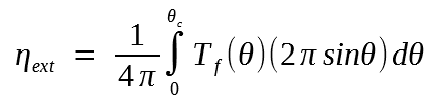where we have assumed that the radiation is emitted uniformly in all directions over a solid angle of 4π. The Fresnel transmissivity Tf depends on the incidence angle θ. In the case of normal incidence (θ = 0), Tf (0) = 4n/(n+1)2. If we replace for simplicity Tf (θ) by Tf (0) in the equation above, ηext is given approximately by

ηext = n-1(n+1)-2

We then obtain the power emitted from one facet. If we use n = 3.5 as a typical value, ηext = 1.4%, indicating that only a small fraction of the internal power becomes the useful output power. A further loss in useful power occurs when the emitted light is coupled into an optical fiber. Because of the incoherent nature of the emitted light, an LED acts as Lambertian source with an angular distribution S(θ) = S0cos(θ), where S0 is the intensity in the direction θ = 0. The coupling efficiency for such a source scales with the numerical aperture (NA) as (NA)2. Since NA for optical fibers falls typically in the range 0.1-0.3, only a few percent of the emitted power is coupled into the fiber (100 μW or less), even though the internal power can easily exceed 10 mW.

A measure of the LED performance is the total quantum efficiency ηtot, defined as the ratio of the emitted optical power Pe to the applied electrical power, Pelec = V0I, where V0 is the voltage drop across the device. ηtot is given by

ηtot =  ηextηint(ћω/qV0)

Typically, ћω ≈ qV0, and ηtot ≈  ηextηint. The total quantum efficiency ηtot, also called the power-conversion efficiency or the wall-plug efficiency, is a measure of the overall performance of the device.

Another quantity sometimes used to characterize the LED performance is the responsivity defined as the ratio RLED = Pe/I. We get

RLED =  ηextηint(ћω/q)

A comparison of the two equations above shows that RLED =  ηtotV0. Typical values of RLED are ~0.01 W/A. The responsivity remains constant as long as the linear relation between Pe and I holds. In practice, this linear relationship holds only over a limited current range. The following figure (a) shows the power-current (P-I) curves at several temperatures for a typical 1.3-μm LED. The responsivity of the device decreases at high currents above 80 mA because of the bending of the P-I curve. One reason for this decrease is related to the increase in the active-region temperature. The internal quantum efficiency ηint is generally temperature-dependent because of an increase in the nonradiative recombination rates at high temperatures.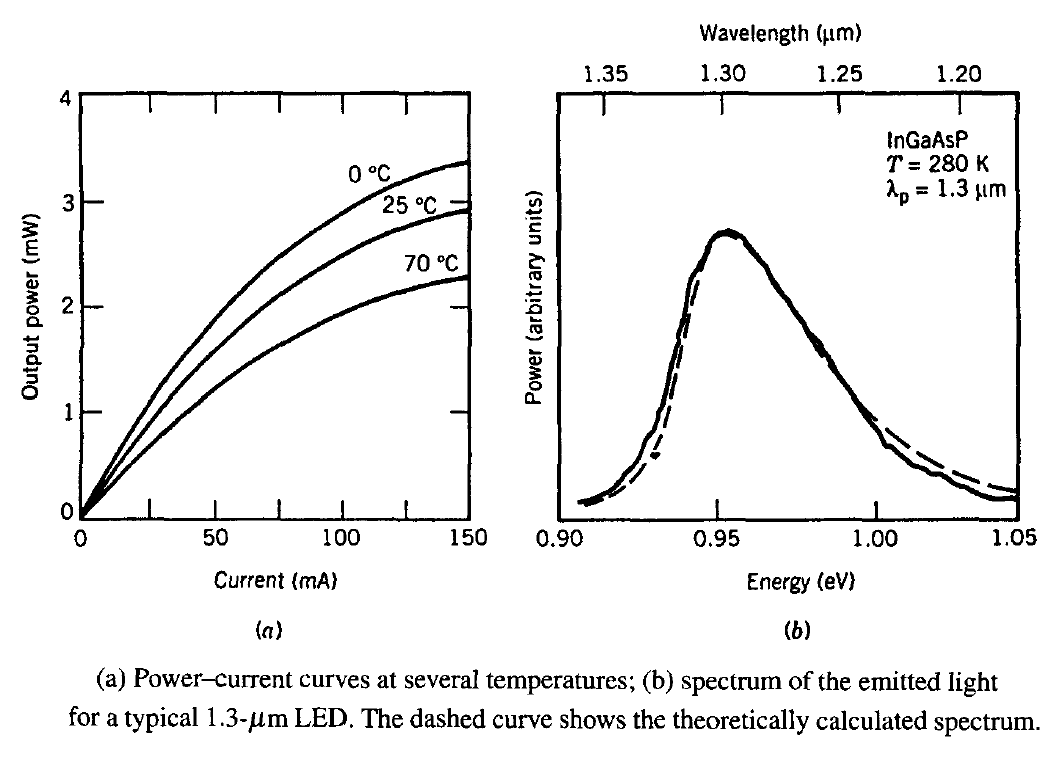The LED spectrum is related to the rate of the spontaneous emission given approximately by

Rspon(ω) = A0ω - Eg)1/2 exp[ - (ћω - Eg)/kBT]

where A0 is a constant, kB is the Boltzmann constant, and Eg is the bandgap. It is easy to deduce that Rspon(ω) peaks when ћω = Eg + kBT/2 and has a full-width at half-maximum (FWHM) Δν ≈ 1.8kBT/h. At room temperature (T = 300 K) the FWHM is about 11 THz. In practice, the spectral width is expressed in nanometers by using Δν = (c/λ2)Δλ and increases as λ2 with an increase in the emission wavelength λ. As a result, Δλ is larger for InGaAsP LEDs emitting at 1.3 μm by about a factor of 1.7 compared with GaAs LEDs. The figure (b) above shows the output spectrum of a typical 1.3-μm LED and compares it with the theoretical curve obtained by using the equation above. Because of a large spectral width (Δλ = 50-60 nm), LEDs are suitable primarily for local-area networks and are often used in combination with plastic fibers to reduce the overall system cost.

#### 2. Modulation Response

The modulation response of LEDs depends on carrier dynamics and is limited by the carrier lifetime τc. It can be determined by using the carrier rate equation from semiconductor lasers after dropping the last term resulting from stimulated emission. The resulting equation isIt can be easily solved in the Fourier domain owing to its linear nature. Consider sinusoidal modulation of the injected current in the form

I(t) = Ib + Imexp(iωmt)

where Ib is the bias current, Im is the modulation current, and ωm is the modulation frequency. We can then solve the equation rate equation above and get N(t) as

N(t) = Nb + Nmexp(iωmt)

where Nb = τcIb/q and Nm is given by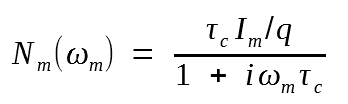The modulated power Pm is related to |Nm| linearly. One can define the LED transfer function H(ωm) as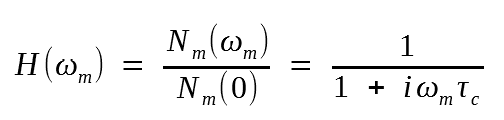The 3-dB modulation bandwidth f3 dB is defined as the frequency at which |H(ωm)| is reduced by 3 dB, a by a factor of 2. The result is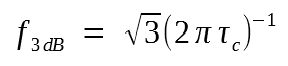Typically, τc is in the range of 2-5 ns for InGaAsP LEDs. The corresponding LED modulation bandwidth is in the range of 50-140 MHz. Note that the equation above provides the optical bandwidth because f3 dB is defined as the frequency at which optical power is reduced by 3 dB. The corresponding electrical bandwidth is the frequency at which |H(ωm)|2 is reduce by 3 dB and is given by (2πτc)-1.

#### 3. LED structures

The LED structures can be classified as surface-emitting or edge-emitting, depending on whether the LED emits light from a surface that is parallel to the junction plane or from the edge of the junction region. Both types can be made using either a p-n homojunction or a heterostructure design in which the active region is surrounded by p- and n-type cladding layers. The heterostructure design leads to superior performance, as it provides a control over the emissive area and eliminates internal absorption because of the transparent cladding layers.

The following figure shows schematically a surface-emitting LED design referred to as the Burrus-type LED. The emissive area of the device is limited to a small region whose lateral dimension is comparable to the fiber-core diameter. The use of a gold stud avoids power loss from the back surface. The coupling efficiency is improved by etching a well and bringing the fiber close to the emissive area. The power coupled into the fiber depends on many parameters, such as the numerical aperture of the fiber and the distance between fiber and LED. The addition of epoxy in the etched well tends to increase the external quantum efficiency as it reduces the refractive-index mismatch. Several variations of the basic design exist in the literature. In one variation, a truncated spherical microlens fabricated inside the etched well is used to couple light into the fiber. In another variation, the fiber end is itself made in the form of a spherical lens. With a proper design, surface-emitting LEDs can couple up to 1% of the internally generated power into an optical fiber.The edge-emitting LEDs employ a design identical to that used for semiconductor lasers. IN fact, a semiconductor laser is converted into an LED by depositing an antireflection coating on its output facet to suppress lasing action. Beam divergence of edge-emitting LEDs differ from surface-emitting LEDs because of waveguiding in the plane perpendicular to the junction. Surface-emitting LEDs operate at as a Lambertian source with angular distribution Se(θ) = S0cosθ in both directions. The resulting beam divergence has a FWHM of 120° in each direction. In contrast, edge-emitting LEDs have a divergence of only about 30° in the direction perpendicular to the junction plane. Considerable light can be coupled into a fiber of even low numerical aperture (<0.3) because of reduced divergence and high radiance at the emitting facet. The modulation bandwidth of edge-emitting LEDs is general alarger (~200 MHz) than that of surface-emitting LEDs because of a reduced carrier lifetime at the same applied current. The choice between the two designs is dictated, in prace, by a compromise between cost and performance.

In spite of a relatively low output power and a low bandwidth of LEDs compared with those of lasers, LEDs are useful for low-cost applications requireing data transmission at a bit rate of 100 Mb/s or less over a few kilometers. For this reason several new LED structures were developed during the 1990s. In one design, known as resonant-cavbity LED, two metal mirrors are fabricated around the epitaxially grown layers, and the device is bonded to a silicon substrate. In a variant of this idea, the bottom mirror is fabricated epitaxially by using a stack of alternating layers of two different semiconductors, while the top mirror consists of a deformable membrane suspended by an air gap. The operating wavelength of such an LED can be tuned over 40 nm by changing the air-gap thickness. In another scheme, several quantum wells with different compositions and bandgaps are grown to form a MQW structure. Since each quantum well emits light at a different wavelength, such LEDs can have an extremely broad spectrum (extending over a 500-nm wavelength range) and are useful for local-area WDM networks.